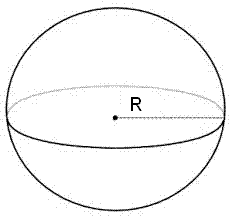# Sphere Calculator

Calculator will calculate the Volume of Sphere and Curved Surface Area(CSA) of Sphere for given radius values.

Volume of Sphere: [ (4/3)πr³ ]
 Volume of Sphere =

Volume of Sphere: [ (4/3)πr³ ]

Curved Surface Area(CSA) of Sphere: [ 4πr² ]

 Curved Surface Area(CSA) of Sphere =### Formula

Volume of Sphere: [ (4/3)πr³ ]

Curved Surface Area(CSA) of Sphere: [ 4πr² ]

In geometry, sphere is a three-dimensional object it has a surface area and volume, sphere is the set of all points in 3D space lying the same distance (R) from a given point (centre), means that the result of rotating a circle about one of its diameters.

The diameter of a sphere is : 2 x radius

The examples of a sphere are football, basketball and model of a globe.